# Comparing decimals

Here you will learn about comparing decimals, including how to compare decimals.

Students will first learn about comparing decimals as a part of number and operations – fractions in 4 th grade and expand upon it in 5 th grade.

## What is comparing decimals?

Comparing decimals is determining which of two or more decimal numbers are greater than, less than or equal. When comparing decimals, you are finding the value of the decimal number, and deciding which one is greater or if they are equal.

You can compare decimal numbers using a place value chart.

For example,

Let’s compare the decimal numbers 0.78 and 0.783.

You will first place the decimal numbers into the place value chart, aligning the numbers by the decimal point.

Start comparing with the largest (left-most) digit, the tenths place. Both numbers have the digit 7 in the tenths place, so move to the next digit in the next place, the hundredths place. Both numbers have the digit 8, so move to the final place, the thousandths place.

0.783 has the digit 3 in the thousandths place and 0.78 doesn’t have a number in the thousandths place, so a zero can be used as a placeholder. 3 is greater than 0, therefore, 0.783 is the greater number and 0.78 is the smaller number.

This can be written as a comparison statement.

0.78<0.783 - 0.78 is less than 0.783, or

0.783>0.78 - 0.783 is greater than 0.78.

The same method can be used when comparing numbers with whole numbers and decimals or ordering decimals in ascending order or descending order. You would just place all decimals being ordered into the place value chart.

### What is comparing decimals?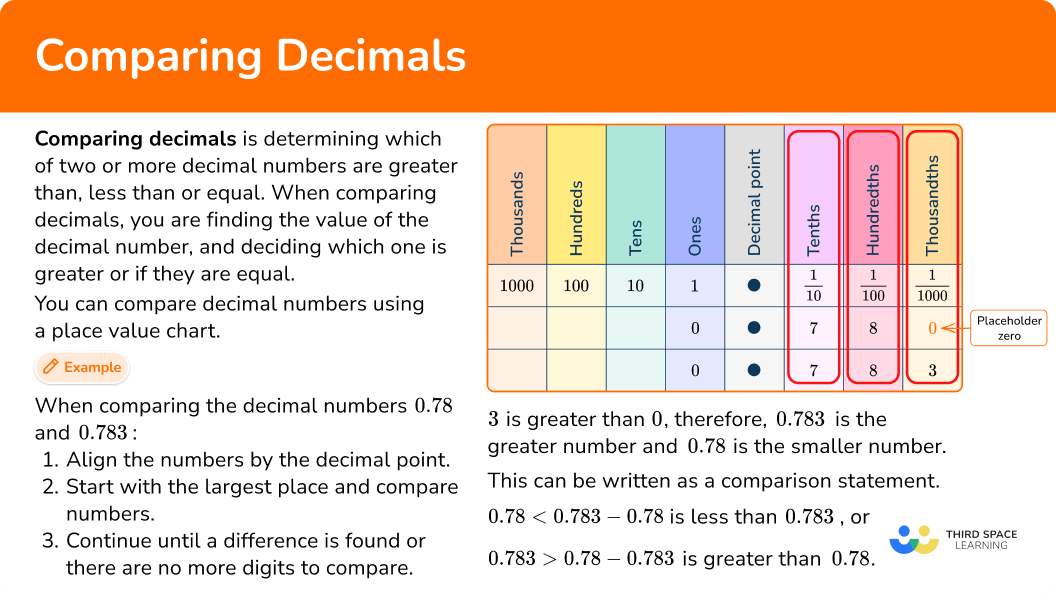## Common Core State Standards

How does this relate to 4 th and 5 th grade math?

• Grade 4: Number and Operations – Fractions (4.NF.C.7)
Compare two decimals to hundredths by reasoning about their size. Recognize that comparisons are valid only when the two decimals refer to the same whole. Record the results of comparisons with the symbols >, =, or <, and justify the conclusions, example, by using a visual model.

• Grade 5: Number and Operations in Base Ten (5.NBT.A.3b)
Compare two decimals to thousandths based on meanings of the digits in each place, using >, =, and < symbols to record the results of comparisons.

## How to compare decimals

In order to compare decimals, you need to:

1. Align the numbers by the decimal point.
3. Continue until a difference is found or there are no more digits to compare.
4. Complete or write a comparison statement using the symbols >, < or =.

## Comparing decimals examples

### Example 1: comparing decimals

Compare the following decimals: 0.65 and 0.46.

1. Align the numbers by the decimal point.

Compare the given decimal numbers, 0.65 and 0.46.

Line up the decimal points using a place value chart if needed.

Start comparing at the largest place, which is the tenths place.

The digits in the tenths place are the same, so compare the digits.

Here you compare the digits 6 and 4. 6 is greater than 4, therefore 0.65 is larger than 0.46.

4Complete or write a comparison statement using the symbols >, < or =.

Write a comparison statement using the correct symbols.

0.65>0.46

### Example 2: comparing decimals

Compare the following decimals: 0.135 and 0.167.

Align the numbers by the decimal point.

Continue until a difference is found or there are no more digits to compare.

Complete or write a comparison statement using the symbols >, < or =.

### Example 3: comparing decimals with whole numbers

Compare the following decimals: 3.456 and 3.018.

Align the numbers by the decimal point.

Continue until a difference is found or there are no more digits to compare.

Complete or write a comparison statement using the symbols >, < or =.

### Example 4: comparing decimals with whole numbers

Compare the following decimals: 104.76 and 104.22.

Align the numbers by the decimal point.

Continue until a difference is found or there are no more digits to compare.

Complete or write a comparison statement using the symbols >, < or =.

### Example 5: comparing decimals (word problem)

Frederick and Samantha are saving money for their summer vacation. Frederick has saved \$27.98 and Samantha has saved \$27.89. Who has saved the greatest amount of money so far?

Align the numbers by the decimal point.

Continue until a difference is found or there are no more digits to compare.

Complete or write a comparison statement using the symbols >, < or =.

### Example 6: comparing decimals (word problem)

Peter is training for the upcoming swimming championship. In week 1, he swam 800 m in 9.324 minutes. In week 2, he swam the same length in 9.243 minutes. Which week did he swim 800 m the fastest?

Align the numbers by the decimal point.

Continue until a difference is found or there are no more digits to compare.

Complete or write a comparison statement using the symbols >, < or =.

### Teaching tips for comparing decimals

• The use of place value charts, number lines, base-ten blocks or decimal tiles will help students compare decimals by allowing them to visualize the value of each place.

• While math worksheets and decimal worksheets have their place for practice, consider using more hands-on activities, like the use of money (pennies and dimes) for students to physically move and compare decimals.

### Easy mistakes to make

• Not lining up the decimal points correctly
When comparing numbers with decimal places, the numbers should be lined up based on the position of their decimal point, not the number of digits.

For example,

• Unfamiliarity with decimal place value
Students that are unfamiliar with how decimal place value works may assume that it works similarly to whole number place value, and that the further the place is from the decimal, the larger the value. However, in decimal place value, the further away the digit is to the right of the decimal, the smaller the place value is.

• Confusing the comparison symbols
Students may mix up the greater than (>), less than (<), and equals (=) symbols.

### Practice comparing decimals questions

1. Which of the following comparison statements correctly compares the decimals 0.76 and 1.23?

0.76>1.231.23<0.760.76<1.230.76=1.23Line up the decimals by the decimal point and begin comparing at the largest place value, the ones place.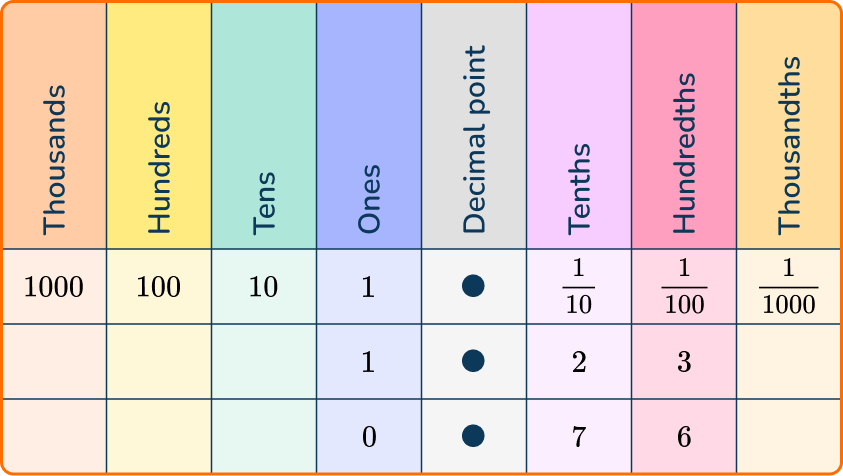The digits in the ones place are different, so compare those digits.

0 is less than 1, so 0.76 is smaller than 1.23.

0.76 is less than 1.23, or 0.76<1.23.

2. Which of the following comparison statements correctly compares the decimals 0.882 and 0.9?

0.882>0.90.882<0.90.882=0.90.9<0.882Line up the decimals by the decimal point and begin comparing at the largest place value, the tenths place.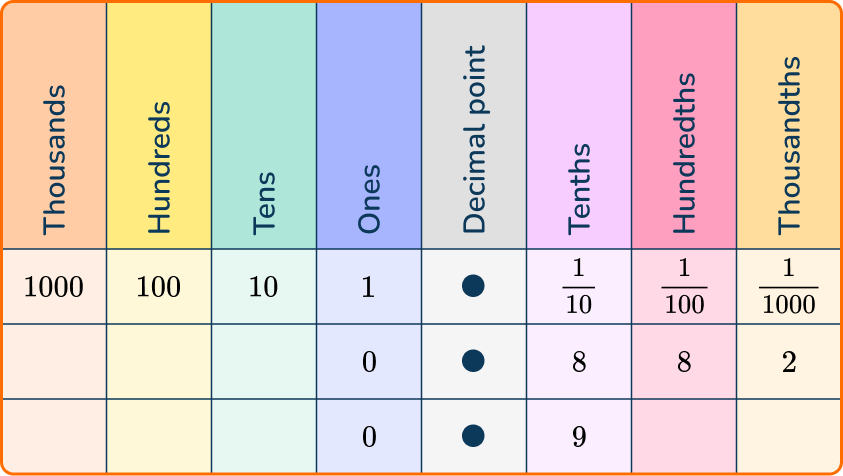The digits in the tenths place are different, so compare those digits.

8 is less than 9, so 0.882 is smaller than 0.9.

0.882 is less than 0.9, or 0.882<0.9.

3. Which of the following comparison statements correctly compares the decimals 23.87 and 23.871?

23.87>23.87123.87=23.87123.871<23.8723.87<23.871Line up the decimals by the decimal point and begin comparing at the largest place value, the tens place.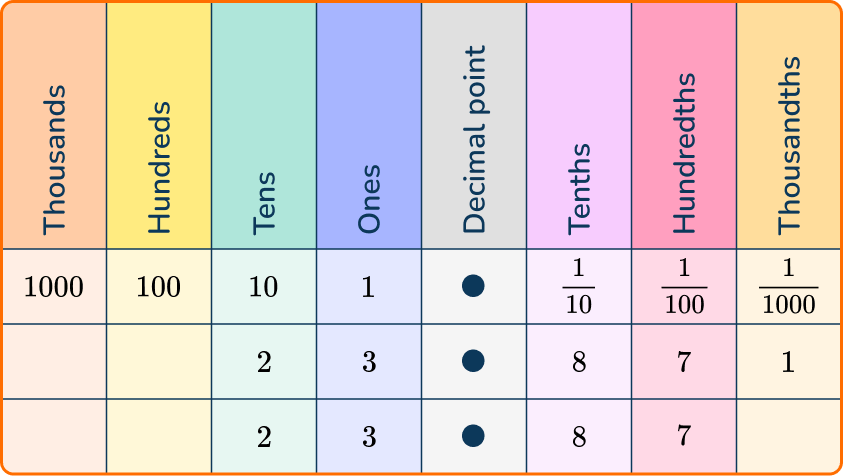The digits in the tens place are not different, so move to the next place value to the right until you find a place where the digits are different. The digits in the thousandths place are different, so compare those digits.

0 is less than 1, so 23.87 is smaller than 23.871.

23.87 is less than 23.871, or 23.87<23.871.

4. Which of the following comparison statements correctly compares the decimals 11.98 and 1.198?

11.98=1.19811.98>1.19811.98<1.1981.198>11.98Line up the decimals by the decimal point and begin comparing at the largest place value, the tens place.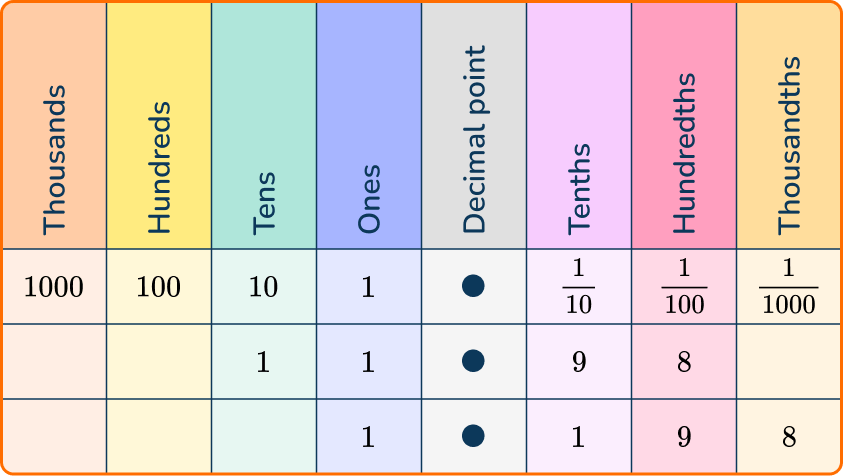The digits in the tens place are different because there is not a digit in the tens place in 1.198, so compare those digits.

0 is less than 1, so 1.198 is smaller than 11.98.

1.198 is less than 11.98, or 1.198<11.98.

5. Ruby spent \$23.17 at the store over the weekend. Cassie spent \$32.71 at the same store. Which comparison statement correctly compares the amount of money Ruby and Cassie spent at the store?

\$32.71 < \$23.17\$32.71 = \$23.17\$32.71 > \$23.17\$23.17 > \$32.71The question is asking you to compare the amounts of money that Cassie and Ruby spent at the mall.

Line up the decimals by the decimal point and begin comparing at the largest place value, the tens place.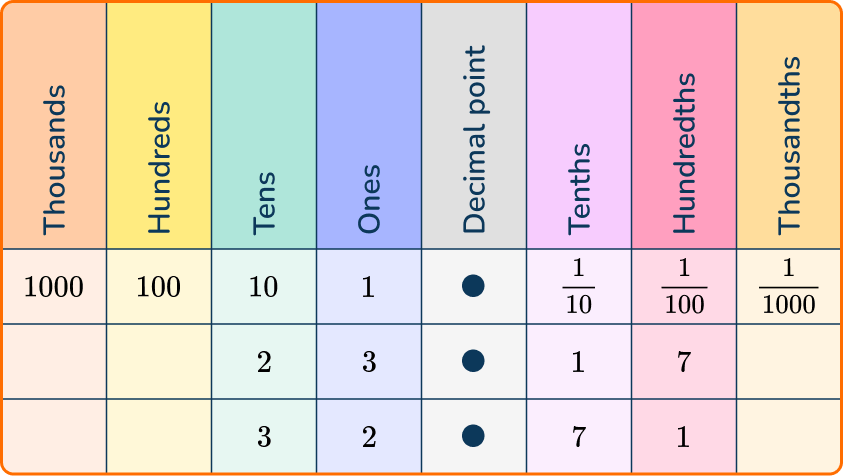The digits in the tens place are different, so compare those digits.

2 is less than 3, so 23.17 is smaller than 32.71.

32.71 is greater than 23.17, or 32.17>23.17.

6. Mrs. Smith’s 4 th graders are taking turns running laps around the blacktop on the playground. The table below shows the times of 4 students.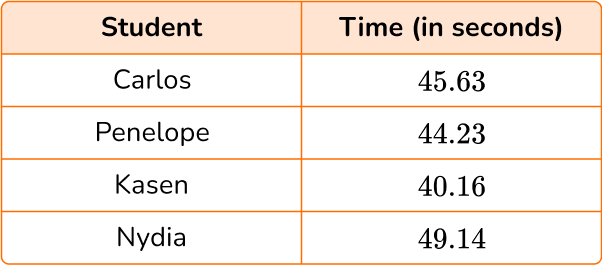Which comparison statement correctly compares the times of Nydia and Carlos?

49.14<45.6344.23<45.6340.16>45.6349.14>45.63The question is asking you to compare the times of Carlos and Nydia. You do not need the other times presented in the word problem.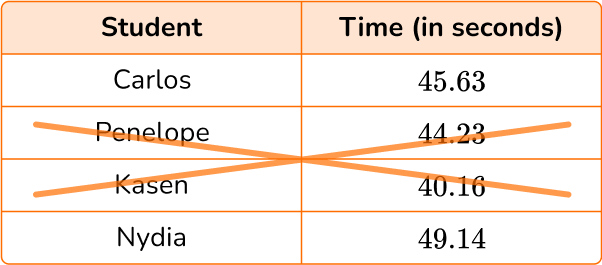Line up the decimals by the decimal point and begin comparing at the largest place value, the tens place.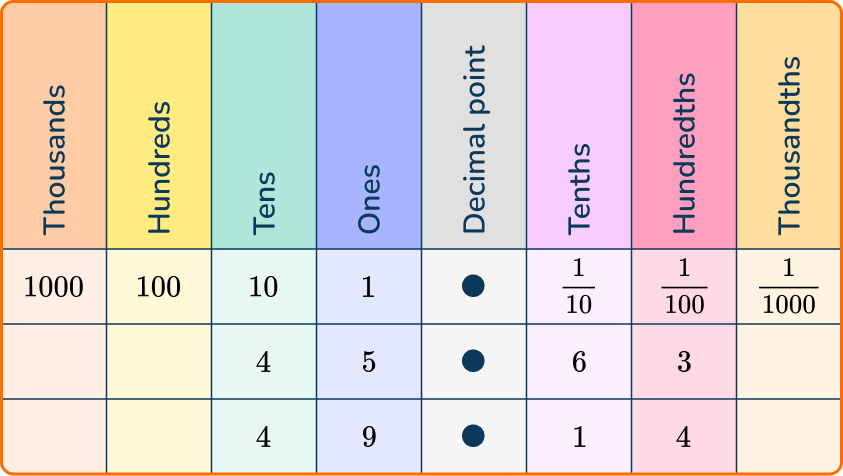The digits in the tens place are not different, so move to the next place value to the right until you find a place where the digits are different. The digits in the ones place are different, so compare those digits.

5 is less than 9, so 45.63 is smaller than 49.14.

45.63 is less than 49.14, or 45.63<49.14.

## Comparing decimals FAQs

What is the difference between comparing numbers and ordering numbers?

Comparing numbers usually involves looking at two numbers and deciding which one is greater or if they’re equal. Ordering is when you are arranging more than 2 numbers or decimals from greatest to least or least to greatest.

How can I compare decimals and fractions?

In order to compare decimals and fractions, you can either convert the fraction to a decimal or convert the decimals to a fraction.

## Still stuck?

At Third Space Learning, we specialize in helping teachers and school leaders to provide personalized math support for more of their students through high-quality, online one-on-one math tutoring delivered by subject experts.

Each week, our tutors support thousands of students who are at risk of not meeting their grade-level expectations, and help accelerate their progress and boost their confidence.